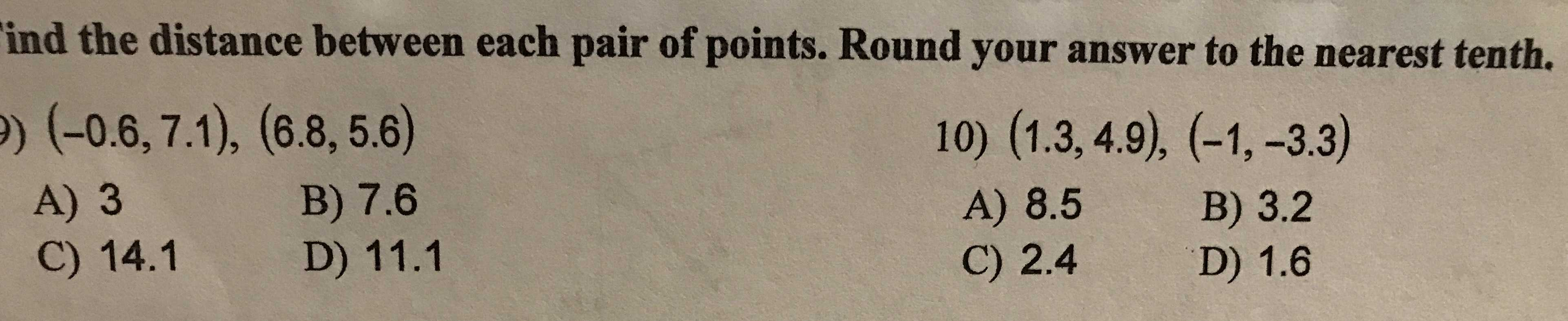### Still have math questions?

Trigonometry
QuestionFind the distance between each pair of points. Round your answer to the nearest tenth. $$( - 0.6,7.1 ) , ( 6.8,5.6 )$$

A) $$3$$ B) $$7.6$$ C) $$14.1$$ D) $$11.1$$ 10) $$( 1.3,4.9 ) , ( - 1 , - 3.3 )$$

A) $$8.5$$ B) $$3.2$$ C) $$2.4$$ D) $$1.6$$